# Discretized Optimal Trajectory, Problem-Based

This example shows how to solve a discretized optimal trajectory problem using the problem-based approach. The trajectory has constant gravity, limits on the applied force, and no air resistance. The solution process solves for the optimal trajectory over a fixed time $T$ and uses that solution to find the optimal $T$, meaning the time that minimizes the cost. The example then shows how to include air resistance, and finally how to include the effect of variable mass as the object consumes fuel.

Compared to a nondiscretized optimization, such as the example Fit ODE Parameters Using Optimization Variables, the discretized version is not as accurate at solving an ordinary differential equation (ODE). However, the discretized version is not affected by noise in the variable-step ODE solver, as described in Optimizing a Simulation or Ordinary Differential Equation. The discretized version can also be easier to customize, and is straightforward to model. Finally, the discretized version can take advantage of automatic differentiation in the optimization process; see Effect of Automatic Differentiation in Problem-Based Optimization.

### Problem Description

The problem is to move an object from position ${p}_{0}$ at time $0$ to position ${p}_{F}$ at time $T$ using a controlled jet. Represent position as a vector $p\left(t\right)$, velocity as a vector $v\left(t\right)$, and applied acceleration as a vector $a\left(t\right)$. In continuous time, the equations of motion, including the force of gravity, are

`$\frac{dp}{dt}=v\left(t\right)$`

$\frac{dv}{dt}=a\left(t\right)+g*\left[0,0,-1\right]$.

The jet applies an acceleration $a\left(t\right)$. The resulting acceleration has an added term for gravity.

The maximum acceleration the jet can apply is ${A}_{max}=25$.

Solve the problem by discretizing time. Divide time into $N$ equal intervals of size $t=T/N$. The position at time step $i$ is a vector $p\left(i\right)$, the velocity is a vector $v\left(i\right)$, and the applied acceleration is a vector $a\left(i\right)$. You can make a set of equations that represent an ODE model fairly accurately. Some approximate equations of motion are

`$v\left(i\right)=v\left(i-1\right)+t\left(a\left(i-1\right)+g\right),\phantom{\rule{0.5em}{0ex}}i=1...N$`

`$p\left(i\right)=p\left(i-1\right)+t\left(\frac{v\left(i-1\right)+v\left(i\right)}{2}\right),\phantom{\rule{0.5em}{0ex}}i=1...N.$`

The preceding equations integrate velocity using a two-point (trapezoidal rule) approximation, and integrate acceleration using a one-point (Euler) approximation. If you regard the acceleration $a\left(i\right)$ as applying to the center of time interval $i$, the integration scheme is a midpoint rule for acceleration. The overall integration scheme gives simple equations: the position and velocity at step $i$ depend only on the position, velocity, and acceleration at step $i-1$. The equations are also easy to modify for air resistance.

The boundary conditions are $p\left(0\right)={p}_{0}$, $p\left(N\right)={p}_{F}$, and $v\left(0\right)=v\left(N\right)=\left[0,0,0\right]$. Set the initial and final positions.

```p0 = [0 0 0]; pF = [5 10 3];```

MATLAB® indices start at 1, not 0. For easier indexing, specify the number of intervals as $N-1$, and have the indices of position and velocity go from $1$ through $N$ instead of $0$ through $N$. With this indexing, the acceleration has indices $1$ through $N-1$.

The cost of using the jet to create force $a$ for time $t$ is $‖a‖t$. The total cost is the sum of the norms of the accelerations times $t$.

`$Cost=\sum _{i=1}^{N-1}‖a\left(i\right)‖t.$`

To convert this cost to a linear cost in optimization variables, create variables $s\left(i\right)$ and associated second-order cone constraints.

`$\begin{array}{l}Cost=\sum _{i=1}^{N-1}s\left(i\right)t\\ ‖a\left(i\right)‖\le s\left(i\right).\end{array}$`

Impose additional constraints that the norm of the acceleration is bounded by a constant `Amax` for all time steps.

`$‖a\left(i\right)‖\le Amax.$`

These constraints are also second-order cone constraints. Because the constraints are linear or second-order cone constraints, and the objective function is linear, `solve` calls the `coneprog` solver to solve the problem.

The `setupproblem1` helper function at the end of this example creates an optimization problem for a fixed time T. The code incorporates the equations of motion as problem constraints. The function includes an air resistance argument; set `air = true` for a model with air resistance. For the definition of air resistance, see the section Include Air Resistance.

The applied acceleration $a$ is the main optimization variable in the problem. As Vanderbei  suggests, the problem does not need to take velocity `v` and position `p` as optimization variables. You can derive their values from the equations of motion and the values of the applied acceleration $a$. For `N` steps, $a$ has dimensions `N``1` by `3`. The problem also includes the vector variable `s` as an optimization variable that allows for a linear objective function.

### Solve Problem for T = 20

Create and solve a trajectory problem for time $T=20$. For reliability in this numerically sensitive problem, set the optimality tolerance to `1e-9`.

`[trajprob,p] = setupproblem1(20);`

What is the default solver for this problem?

`defaultsolver = solvers(trajprob)`
```defaultsolver = "coneprog" ```

Set the options for the `coneprog` solver and solve the problem.

```opts = optimoptions(defaultsolver,Display="none",OptimalityTolerance=1e-9); [sol,fval,eflag,output] = solve(trajprob,Options=opts)```
```sol = struct with fields: a: [49×3 double] s: [49×1 double] ```
```fval = 192.2812 ```
```eflag = OptimalSolution ```
```output = struct with fields: iterations: 12 primalfeasibility: 4.4823e-10 dualfeasibility: 3.3916e-09 dualitygap: 3.2421e-11 algorithm: 'interior-point' linearsolver: 'augmented' message: 'Optimal solution found.' solver: 'coneprog' ```

Plot the trajectory and norm of the acceleration by calling the `plottrajandaccel` helper function.

```N = 50; plottrajandaccel(sol,p,p0,pF)```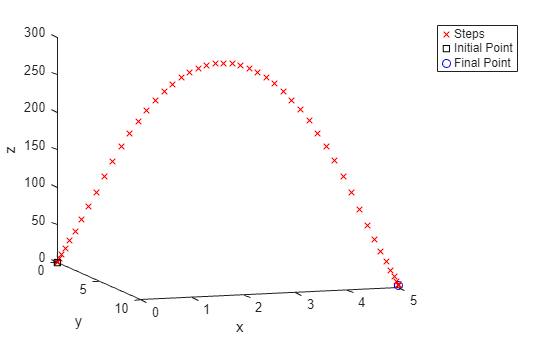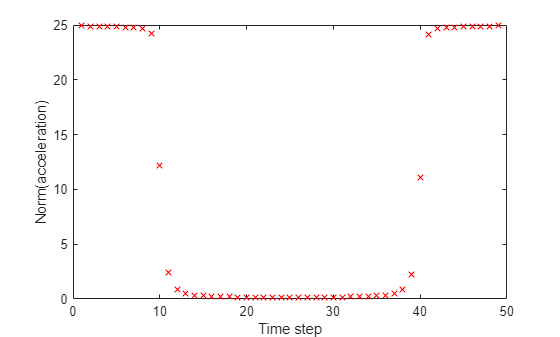The acceleration is nearly maximal at the beginning and end periods, and nearly zero in the middle. This type of solution, where acceleration is either maximal or 0, is called "bang-bang."

### Find Minimal Cost

What time $T$ causes the cost to be minimal? For too small a time, such as $T=1$, the problem is infeasible, meaning it has no solution.

```myprob = setupproblem1(1); opts.Display = "final"; % To see the solution status [solm,fvalm,eflagm,outputm] = solve(myprob,Options=opts);```
```Solving problem using coneprog. Problem is infeasible. ```

Time 1.5 gives a feasible problem.

```myprob = setupproblem1(1.5); [solm,fvalm,eflagm,outputm] = solve(myprob,Options=opts);```
```Solving problem using coneprog. Optimal solution found. ```

The `tomin` helper function sets up a problem for time `T` and then calls `solve` to calculate the cost of the solution. Call `fminbnd` on `tomin` to find the optimal time (lowest cost possible) in the interval $1.5\le T\le 10$.

`[Tmin,Fmin] = fminbnd(@(T)tomin(T,false),1.5,10)`
```Tmin = 1.9517 ```
```Fmin = 24.6095 ```

Obtain the trajectory for the optimal time `Tmin`.

```[minprob,p] = setupproblem1(Tmin); sol = solve(minprob,Options=opts);```
```Solving problem using coneprog. Optimal solution found. ```

Plot the minimizing trajectory and acceleration.

`plottrajandaccel(sol,p,p0,pF)`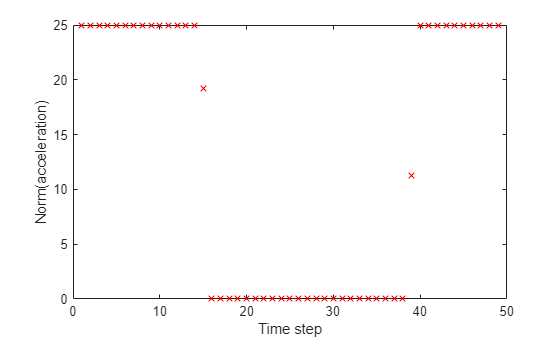The minimizing solution is closer to a "bang-bang" solution: the acceleration is either maximal or zero for all but two values.

### Plot Nonminimizing Trajectories

Plot the trajectories for a variety of times.

```figure hold on options = optimoptions("coneprog",Display="none",OptimalityTolerance=1e-9); g = [0 0 -9.81]; for i = 1:10 T = 2*i; t = T/N; prob = setupproblem1(T); sol = solve(prob,"Options",options); asol = sol.a; vsol = cumsum([[0 0 0];t*(asol+repmat(g,size(asol,1),1))]); N = size(vsol,1); np = 2:N; n0 = 1:(N-1); psol = cumsum([p0;t*(vsol(np,:) + vsol(n0,:))/2]); plot3(psol(:,1),psol(:,2),psol(:,3),'rx',"Color",[256-25*i 20*i 25*i]/256) end view([18 -10]) xlabel("x") ylabel("y") zlabel("z") legend("T = 2","T = 4","T = 6","T = 8","T = 10","T = 12","T = 14","T = 16","T = 18","T = 20") hold off```The shortest time (2) has a nearly direct trajectory on this scale. Longer times have increasingly large variations from a direct path, with the path for $T=20$ reaching a height near 300.

### Include Air Resistance

Change the model dynamics to include air resistance. Linear air resistance changes the velocity by a factor $\mathrm{exp}\left(-t\right)$ after time $t$. The equations of motion become

`$v\left(i\right)=v\left(i-1\right)\mathrm{exp}\left(-t\right)+t\left(a\left(i-1\right)+g\right)$`

`$\begin{array}{l}p\left(i\right)=p\left(i-1\right)+t\left(\frac{v\left(i-1\right)+v\left(i\right)}{2}\right)\\ \phantom{\rule{1em}{0ex}}\phantom{\rule{1em}{0ex}}=p\left(i-1\right)+t\left(\frac{1+\mathrm{exp}\left(-t\right)}{2}\right)v\left(i-1\right)+{t}^{2}\frac{a\left(i-1\right)+g}{2}.\end{array}$`

The problem formulation in the `setupproblem1(T,air)` function for `air = true` has factors of `exp(-t)` in both the line defining the velocity constraint and the line defining the position constraint:

```vcons(2:N,:) = v(2:N,:) == v(1:(N-1),:)*exp(-t) + t*(a(1:(N-1),:) + repmat(g,N-1,1)); pcons(2:N,:) = p(2:N,:) == p(1:(N-1),:) + t*(1+exp(-t))/2*v(1:(N-1),:) + t^2/2*(a(1:(N-1),:) + repmat(g,N-1,1)); ```

Find the optimal time for the problem with air resistance.

`[Tmin2,Fmin2] = fminbnd(@(T)tomin(T,true),1.5,10)`
```Tmin2 = 1.9398 ```
```Fmin2 = 28.7967 ```

The optimal time is only slightly lower than the time in the problem without air resistance (1.94 compared to 1.95), but the cost `Fmin` is about 17% higher (28.8 compared to 24.6).

`compartable = table([Tmin;Tmin2],[Fmin;Fmin2],'VariableNames',["Time" "Cost"],'RowNames',["Original" "Air Resistance"])`
```compartable=2×2 table Time Cost ______ ______ Original 1.9517 24.609 Air Resistance 1.9398 28.797 ```

Plot the trajectory and acceleration for the optimal solution with air resistance.

```[minprob,p] = setupproblem1(Tmin2,true); sol = solve(minprob,Options=opts);```
```Solving problem using coneprog. Optimal solution found. ```
`plottrajandaccel(sol,p,p0,pF)`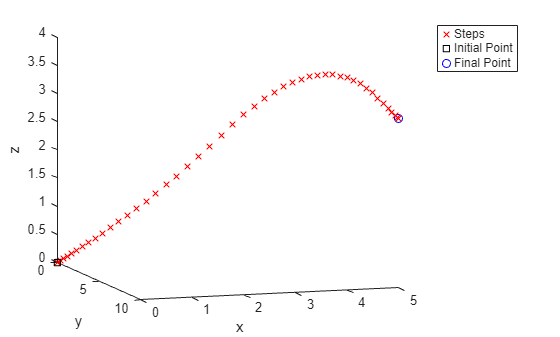With air resistance, the time of zero acceleration shifts to a later time step and is shorter. However, the solution is still nearly "bang-bang."

### Extend Model to Include Variable Mass

A jet operates by expelling mass. If you include the effect of diminishing mass as the jet operates, the equations of motion no longer fit into the framework of a second-order cone problem. The problem becomes harder to solve numerically, leading to longer solution times and less accurate answers.

Change the model to include an applied force $F\left(t\right)$ and a mass $m\left(t\right)$. The net acceleration is

$a\left(t\right)=F\left(t\right)/m\left(t\right)+g\left[0,0,-1\right]$.

The rate of change of mass is

`$\frac{dm}{dt}=-r‖F\left(t\right)‖,$`

where $r$ is a constant.

Assume that $m\left(0\right)=2$, $r=1/1000$, and the maximum force is ${F}_{max}=50$. These assumptions imply that, at time 0, the maximum applied acceleration is ${F}_{max}/m\left(0\right)=25$, the same maximum acceleration as in the previous model.

The `setupproblemfuel1` function creates a problem based on these equations and parameters. Do not include air resistance for this instance of the variable mass model.

`[trajprob,p] = setupproblemfuel1(20);`

What is the default solver for the resulting problem?

`defaultsolver = solvers(trajprob)`
```defaultsolver = "fmincon" ```

The `fmincon` solver requires an initial point. Try a random initial point for T = 20.

```rng default y0 = randn(49,3); x0.F = y0;```

Specify options for the solver to produce plots as it progresses and to allow for more function evaluations and iterations than the default. Try to obtain more accuracy by reducing the `StepTolerance` from the default `1e-10` to `1e-11`.

```opts = optimoptions(defaultsolver,Display="none",PlotFcn="optimplotfvalconstr",... MaxFunctionEvaluations=1e5,MaxIterations=1e4,StepTolerance=1e-11);```

Call the solver.

`[sol,fval,eflag,output] = solve(trajprob,x0,Options=opts)````sol = struct with fields: F: [49×3 double] ```
```fval = 348.1493 ```
```eflag = StepSizeBelowTolerance ```
```output = struct with fields: iterations: 2393 funcCount: 8148 constrviolation: 2.7960e-12 stepsize: 1.8893e-11 algorithm: 'interior-point' firstorderopt: 0.0719 cgiterations: 76125 message: 'Local minimum possible. Constraints satisfied.↵↵fmincon stopped because the size of the current step is less than↵the value of the step size tolerance and constraints are ↵satisfied to within the value of the constraint tolerance.↵↵<stopping criteria details>↵↵Optimization stopped because the relative changes in all elements of x are↵less than options.StepTolerance = 1.000000e-11, and the relative maximum constraint↵violation, 1.474630e-15, is less than options.ConstraintTolerance = 1.000000e-06.' bestfeasible: [1×1 struct] objectivederivative: "reverse-AD" constraintderivative: "reverse-AD" solver: 'fmincon' ```

Plot the trajectory and acceleration. The `plottrajandaccel` function uses the values in the `a` field of the solution structure, so first copy the solution from the `F` field to the `a` field.

```sol.a = sol.F; plottrajandaccel(sol,p,p0,pF)```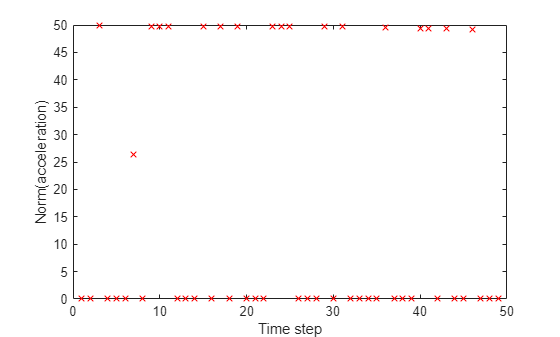### Smooth Solution by Adding Cost for Variable Acceleration

The applied force is essentially "bang-bang," yet the force has oscillatory behavior. Vanderbei  calls this type of behavior "ringing." To remove the ringing, add some cost for oscillatory acceleration, and then solve again. The `setupproblemfuel2` helper function includes an additional term `t*1e-4*sum(diff(fnorm).^2` in the objective function, which penalizes changes in the norm of the acceleration:

``` % Add cost for acceleration changes trajectoryprob.Objective = sum(fnorm)*t + t*1e-4*sum(diff(fnorm).^2); ```

Solve the problem again.

```[trajprob,p] = setupproblemfuel2(20); [sol,fval,eflag,output] = solve(trajprob,x0,Options=opts)``````sol = struct with fields: F: [49×3 double] ```
```fval = 348.5616 ```
```eflag = StepSizeBelowTolerance ```
```output = struct with fields: iterations: 3755 funcCount: 7927 constrviolation: 3.2683e-11 stepsize: 1.6872e-10 algorithm: 'interior-point' firstorderopt: 0.1728 cgiterations: 46335 message: 'Local minimum possible. Constraints satisfied.↵↵fmincon stopped because the size of the current step is less than↵the value of the step size tolerance and constraints are ↵satisfied to within the value of the constraint tolerance.↵↵<stopping criteria details>↵↵Optimization stopped because the relative changes in all elements of x are↵less than options.StepTolerance = 1.000000e-11, and the relative maximum constraint↵violation, 1.723720e-14, is less than options.ConstraintTolerance = 1.000000e-06.' bestfeasible: [1×1 struct] objectivederivative: "reverse-AD" constraintderivative: "reverse-AD" solver: 'fmincon' ```

Plot the trajectory and acceleration using the `sol.F` data.

```sol.a = sol.F; plottrajandaccel(sol,p,p0,pF)```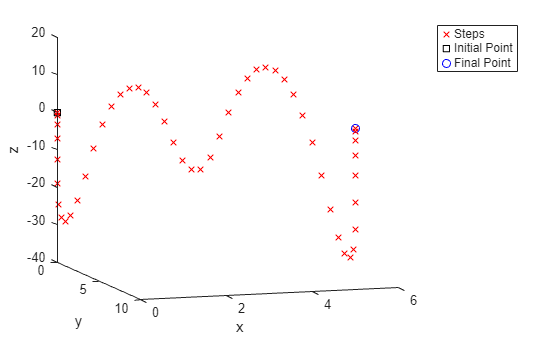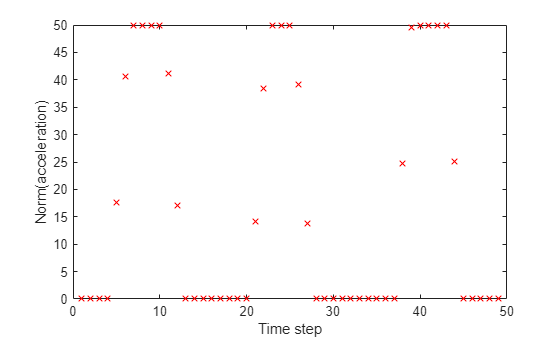### Supply Better Initial Point

Most of the ringing is gone, but the solution still has more than one interval of zero force.

Specifying a different initial point might result in a more satisfactory solution. To cause the solver to have small acceleration during intermediate times, specify the initial point equal to 0 for indices 10 through 40, and equal to 20 for indices 1 through 9 and 41 through 49. Add some random noise to the initial point.

```rng default y0 = 20*ones(49,3); y0(10:40,:) = 0; y0 = y0 + randn(size(y0)); x0.F = y0;```

Solve the problem starting from this new point.

`[sol,fval,eflag,output] = solve(trajprob,x0,Options=opts)````sol = struct with fields: F: [49×3 double] ```
```fval = 348.2770 ```
```eflag = StepSizeBelowTolerance ```
```output = struct with fields: iterations: 1653 funcCount: 5422 constrviolation: 7.6383e-14 stepsize: 2.2832e-11 algorithm: 'interior-point' firstorderopt: 0.0318 cgiterations: 26724 message: 'Local minimum possible. Constraints satisfied.↵↵fmincon stopped because the size of the current step is less than↵the value of the step size tolerance and constraints are ↵satisfied to within the value of the constraint tolerance.↵↵<stopping criteria details>↵↵Optimization stopped because the relative changes in all elements of x are↵less than options.StepTolerance = 1.000000e-11, and the relative maximum constraint↵violation, 6.578331e-17, is less than options.ConstraintTolerance = 1.000000e-06.' bestfeasible: [1×1 struct] objectivederivative: "reverse-AD" constraintderivative: "reverse-AD" solver: 'fmincon' ```

Plot the trajectory and acceleration.

```sol.a = sol.F; plottrajandaccel(sol,p,p0,pF)```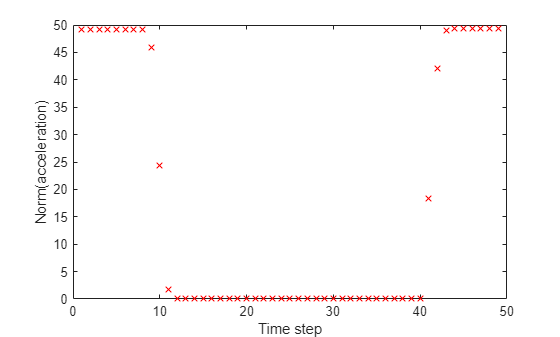The solution seems satisfactory: it is essentially "bang-bang" acceleration with just one interval of zero applied acceleration.

How much mass remains from the initial 2 at the end of the object's journey?

```T = 20; N = 50; t = T/N; fnorm = zeros(N-1,1); r = 1/1000; for i = 1:length(fnorm) fnorm(i) = norm(sol.F(i,:)); end m = 2 - r*t*sum(fnorm)```
```m = 1.6518 ```

What is the maximum applied acceleration with this reduced mass?

```Fmax = 50; Fmax/m```
```ans = 30.2700 ```

When the model includes the effect of variable mass, the maximum applied acceleration during the trajectory changes from 25 to 30.

### Include Air Resistance and Variable Mass

Include air resistance in the model again. In this case, the `setupproblemfuel2` helper function calls the `airResistance` helper function using `fcn2optimexpr` to evaluate the velocity with air resistance. Coding a `for` loop using a separate function, and calling that function using `fcn2optimexpr`, creates a more efficient problem. For more information, see Create for Loop for Static Analysis and Static Analysis of Optimization Expressions.

`[trajprob2,p] = setupproblemfuel2(20,true);`

Based on the result of the earlier model that included air resistance, set the initial interval of zero force to be later: 15 through 45 instead of 10 through 40.

```rng default y0 = 20*ones(49,3); y0(15:45,:) = 0; y0 = y0 + randn(size(y0)); x0.F = y0;```

Solve the problem.

`[sol2,fval2,eflag2,output2] = solve(trajprob2,x0,Options=opts)````sol2 = struct with fields: F: [49×3 double] ```
```fval2 = 352.7138 ```
```eflag2 = StepSizeBelowTolerance ```
```output2 = struct with fields: iterations: 6686 funcCount: 12620 constrviolation: 2.1938e-13 stepsize: 1.5226e-11 algorithm: 'interior-point' firstorderopt: 0.2178 cgiterations: 93737 message: 'Local minimum possible. Constraints satisfied.↵↵fmincon stopped because the size of the current step is less than↵the value of the step size tolerance and constraints are ↵satisfied to within the value of the constraint tolerance.↵↵<stopping criteria details>↵↵Optimization stopped because the relative changes in all elements of x are↵less than options.StepTolerance = 1.000000e-11, and the relative maximum constraint↵violation, 1.532271e-15, is less than options.ConstraintTolerance = 1.000000e-06.' bestfeasible: [1×1 struct] objectivederivative: "reverse-AD" constraintderivative: "reverse-AD" solver: 'fmincon' ```
```sol2.a = sol2.F; plottrajandaccel(sol2,p,p0,pF)```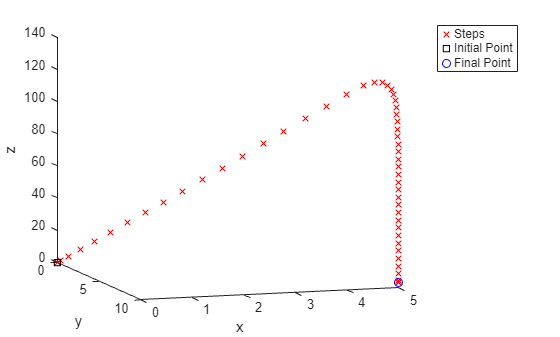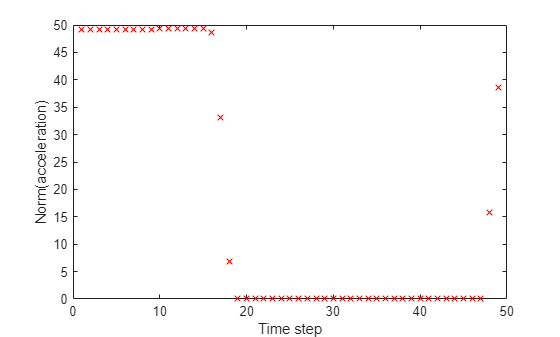What is the mass of the remaining fuel?

```for i = 1:length(fnorm) fnorm(i) = norm(sol2.F(i,:)); end m2 = 2 - r*t*sum(fnorm)```
```m2 = 1.6474 ```

What is the maximum applied acceleration with this reduced mass?

`Fmax/m2`
```ans = 30.3517 ```

The inclusion of air resistance does not change the fuel usage significantly. The trajectory seems to use air resistance to help slow the object, so the object does not use much fuel for deceleration during the final portion of the journey. Notice that the maximum height of the trajectory with air resistance is under 130, but the maximum height without air resistance is about 300. With the current parameters, air resistance makes a significant difference in the trajectory, but not in fuel usage.

### References

 Vanderbei, R. J. "Case Studies in Trajectory Optimization: Trains, Planes, and Other Pastimes." Optimization and Engineering 2, 215–243 (2001). Available at https://vanderbei.princeton.edu/tex/trajopt/trajopt.pdf.

### Helper Functions

This code creates the `setupproblem1` helper function.

```function [trajectoryprob,p] = setupproblem1(T,air) if nargin == 1 air = false; end N = 50; g = [0 0 -9.81]; p0 = [0 0 0]; pF = [5 10 3]; Amax = 25; t = T/N; a = optimvar("a",N-1,3,"LowerBound",-Amax,"UpperBound",Amax); v = optimexpr(N,3); trajectoryprob = optimproblem; s = optimvar("s",N-1,"LowerBound",0,"UpperBound",3*Amax); trajectoryprob.Objective = sum(s)*t; scons = optimconstr(N-1); for i = 1:(N-1) scons(i) = norm(a(i,:)) <= s(i); end acons = optimconstr(N-1); for i = 1:(N-1) acons(i) = norm(a(i,:)) <= Amax; end np = 2:N; n0 = 1:(N-1); v0 = [0 0 0]; if air v(1, :) = v0; for i = 2:N v(i,:) = v(i-1,:)*exp(-t) + t*(a(i-1,:) + g); end else gbig = repmat(g,size(a,1),1); v = cumsum([v0; t*(a + gbig)]); end p = cumsum([p0;t*(v(np,:) + v(n0,:))/2]); % Independent of "air" endcons = v(N,:) == [0 0 0]; pcons2 = p(N,:) == pF; trajectoryprob.Constraints.acons = acons; trajectoryprob.Constraints.scons = scons; trajectoryprob.Constraints.endcons = endcons; trajectoryprob.Constraints.pcons2 = pcons2; end```

This code creates the `plottrajandaccel` helper function.

```function plottrajandaccel(sol,p,p0,pF) figure psol = evaluate(p, sol); plot3(psol(:,1),psol(:,2),psol(:,3),'rx') hold on plot3(p0(1),p0(2),p0(3),'ks') plot3(pF(1),pF(2),pF(3),'bo') hold off view([18 -10]) xlabel("x") ylabel("y") zlabel("z") legend("Steps","Initial Point","Final Point") figure asolm = sol.a; nasolm = sqrt(sum(asolm.^2,2)); plot(nasolm,"rx") xlabel("Time step") ylabel("Norm(acceleration)") end```

This code creates the `tomin` helper function.

```function F = tomin(T,air) if nargin == 1 air = false; end problem = setupproblem1(T,air); opts = optimoptions("coneprog",Display="none",OptimalityTolerance=1e-9); [~,F] = solve(problem,"Options",opts); end```

This code creates the `setupproblemfuel1` helper function.

```function [trajectoryprob,p] = setupproblemfuel1(T,air) r = 1/1000; if nargin == 1 air = false; end N = 50; g = [0 0 -9.81]; p0 = [0 0 0]; pF = [5 10 3]; Fmax = 50; t = T/N; F = optimvar("F",N-1,3,"LowerBound",-Fmax,"UpperBound",Fmax); v = optimexpr(N,3); fnorm = optimexpr(N-1); for i = 1:length(fnorm) fnorm(i) = norm(F(i,:)); end m = 2 - r*t*cumsum(fnorm); trajectoryprob = optimproblem; trajectoryprob.Objective = sum(fnorm)*t; Fcons = fnorm <= Fmax; v0 = [0 0 0]; if air v = fcn2optimexpr(@airResistance, v, v0, N, t, F, m, g); else gbig = repmat(g,size(F,1),1); mbig = repmat(m,1,3); v = cumsum([v0; t*(F./mbig + gbig)]); end np = 2:N; n0 = 1:(N-1); p = cumsum([p0;t*(v(np,:) + v(n0,:))/2]); % Independent of "air" endcons = v(N,:) == [0 0 0]; pcons2 = p(N,:) == pF; trajectoryprob.Constraints.Fcons = Fcons; trajectoryprob.Constraints.endcons = endcons; trajectoryprob.Constraints.pcons2 = pcons2; end```

This code creates the `setupproblemfuel2` helper function.

```function [trajectoryprob,p] = setupproblemfuel2(T,air) r = 1/1000; if nargin == 1 air = false; end N = 50; g = [0 0 -9.81]; p0 = [0 0 0]; pF = [5 10 3]; Fmax = 50; t = T/N; F = optimvar("F",N-1,3,"LowerBound",-Fmax,"UpperBound",Fmax); v = optimexpr(N,3); fnorm = optimexpr(N-1); for i = 1:length(fnorm) fnorm(i) = norm(F(i,:)); end m = 2 - r*t*cumsum(fnorm); trajectoryprob = optimproblem; % Add cost for acceleration changes trajectoryprob.Objective = sum(fnorm)*t + t*1e-4*sum(diff(fnorm).^2); Fcons = fnorm <= Fmax; v0 = [0 0 0]; if air v = fcn2optimexpr(@airResistance, v, v0, N, t, F, m, g); else gbig = repmat(g,size(F,1),1); mbig = repmat(m,1,3); v = cumsum([v0; t*(F./mbig + gbig)]); end np = 2:N; n0 = 1:(N-1); p = cumsum([p0;t*(v(np,:) + v(n0,:))/2]); % Independent of "air" endcons = v(N,:) == [0 0 0]; pcons2 = p(N,:) == pF; trajectoryprob.Constraints.Fcons = Fcons; trajectoryprob.Constraints.endcons = endcons; trajectoryprob.Constraints.pcons2 = pcons2; end```

This code creates the `airResistance` helper function, which is used in `setupproblemfuel1` and `setupproblemfuel2`.

```function v = airResistance(v, v0, N, t, F, m, g) v(1, :) = v0; for i = 2:N v(i,:) = v(i-1,:)*exp(-t) + t*(F(i-1,:)/m(i-1) + g); end end```